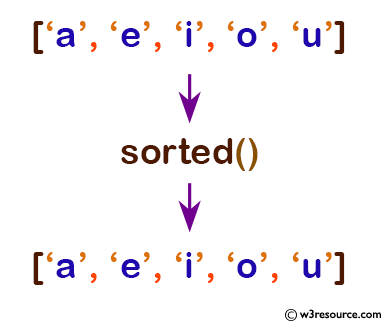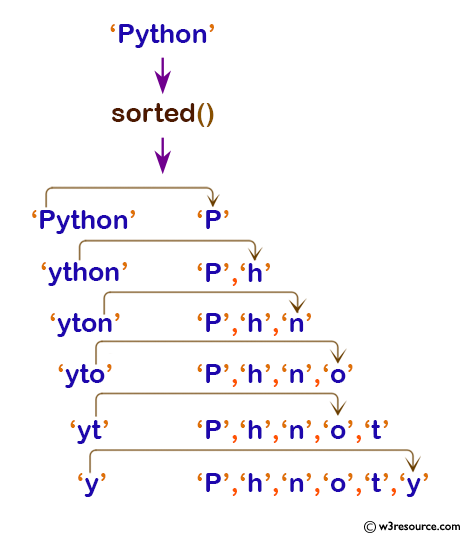# Python: sorted() function

## sorted() function

The sorted() function is used to get a new sorted list from the items in iterable.

Version:

(Python 3.2.5)

Syntax:

```sorted(iterable[, key][, reverse])
```

Parameter:

Name Description Required /
Optional
iterable

The sequence to sort list, dictionary, tuple or collection etc.

Required.
key A function that serves as a key for the sort comparison. Optional.
reverse True will sort descending and default is False Optional.

Return value:

A sorted list from the given iterable.

Example-1: Python sorted() function

``````# vowels list
pyList = ['a', 'e', 'i', 'o', 'u']
print(sorted(pyList))

# string
pyStr = 'Python'
print(sorted(pyStr))

# vowels tuple
pyTuple = ('a', 'e', 'i', 'o', 'u')
print(sorted(pyTuple))
``````

Output:

```['a', 'e', 'i', 'o', 'u']
['P', 'h', 'n', 'o', 't', 'y']
['a', 'e', 'i', 'o', 'u']
```

Pictorial Presentation:Pictorial Presentation:Example-2: Python sorted() with key function

``````# take third element for sort
def takeThird(elem):
return elem

# random list
random = [(2, 2), (3, 4), (4, 1), (1, 3)]

# sort list with key
sortList = sorted(random, key=takeThird)

# print list
print('Sorted list:', sortList)
``````

Output:

```Sorted list: [(4, 1), (2, 2), (1, 3), (3, 4)]
```

Python Code Editor:

Previous: slice()
Next: str()

Test your Python skills with w3resource's quiz

﻿

## Python: Tips of the Day

How do I check if a list is empty?

For example, if passed the following:

```a = []
if not a:
print("List is empty")
```

Ref: https://bit.ly/2A4JXx9